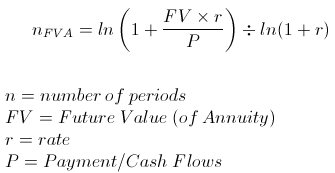I need to create a python script to solve this equation.The code I have written so far is:

```import math
p = 100
r = 0.06 / 12
FV = 4000

n = str(ln * ((1 + (FV * r) / p) / (ln * (1 + r))))

print ("Number of periods = " + str(n))```

The answer I should get is 36.55539635919235. Can someone help me with this?Apr 25 in Python 72 views

## 1 answer to this question.

`n = math.log((1 + (FV * r) / p) / math.log(1 + r)))`

I hope this helps.answered Apr 25 by
• 10,840 points

## How to print square of first 100 natural numbers using iterations in python?

Can you make a program with nested ...READ MORE

## How can I rename a file in Python?

yes, you can use "os.rename" for that. ...READ MORE

## How can I create a new file in Python?

You can try the below code which ...READ MORE

## what is the practical use of polymorphism in Python?

Polymorphism is the ability to present the ...READ MORE

Python doesn't know what \$FILEDIR is. Try an absolute path ...READ MORE

## Iterating over dictionaries using 'for' loops

key is just a variable name. for key ...READ MORE

## Conflicting dependencies of pypyodbc and blpapi

I figured out that pypyodbc only works ...READ MORE

## how do i change string to a list?

suppose you have a string with a ...READ MORE